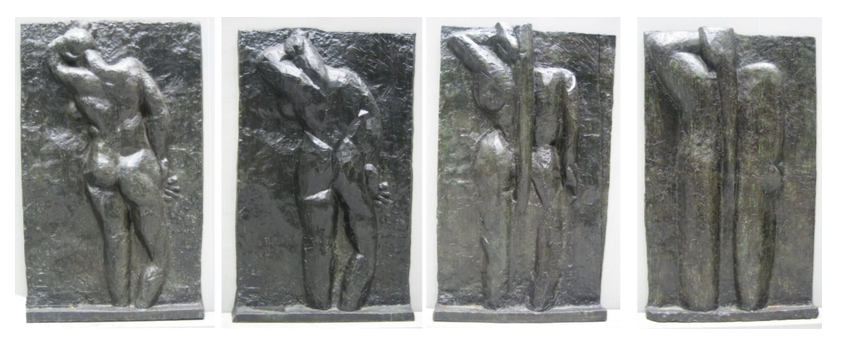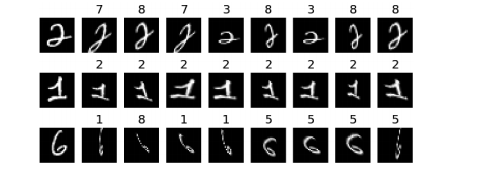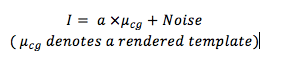# Magic behind “A probabilistic framework for deep learning” explained

Image classification problems can be dealt with from a probabilistic perspective, as discussed in the research paper titled “A Probabilistic Framework for Deep Learning” (which we will simply call “the paper”).

The maths behind this framework is convoluted and might even come across as sorcery (it is not). Keeping in mind that not everyone has maths background, we will use a heuristic approach to gain an intuitive sense of what’s going on. As such, we will not delve into detailed mathematical derivations or technical jargons — math fanatics be warned!

### The DRMM

The paper discusses an image generator called the Deep Rendering Mixture Model (DRMM). It consists of several layers (as implied by the word “Deep”), and in each layer it makes an abstract image more concrete. Here’s an example of it in action:The image rendering process: From right to left, we could see finer details of the sculpture. Picture taken from here.

The above images show four layers of a DRMM. Starting from the right most image at layer L, it renders the image to become more concrete at each successive layer (L-1, L-2…).

### The RMM

A simple, shallow version of the DRMM is the RMM (Rendering Mixture Model), which consists of only one layer. We can think of it as something that renders (fills in) a blank paper with an image. How the RMM performs the rendering depends on class of the image, c and nuisance variables g.

Nuisance variables are variables that are not intended to be part of the classification problem. However, we have to consider them as they cause variations between images. Examples of nuisance variables include geometric distortions, posture and lighting of image.Leftmost column represents original MNIST images, following columns are data transformed by nuisance variables. This is an actual result of an experiment. The predicted labels are the numbers above the nuisance-added images.

Furthermore, the RMM divides this blank paper into many patches, and renders one patch at a time. The RMM can decide whether or not to render a particular patch. This decision is represented by switching variable a = {0,1}.

This rendering process produces a rendered template. Formally, for each patch we have the following:Equation B: Consider cases when a = 0 and a = 1 to infer which value corresponds to a decision to render a patch.

Therefore, to an RMM, an image I is characterised by three variables:

1. Class variables c : Image categories
2. Nuisance variables g: Factors that cause images to vary
3. Switching variable a: Decision whether or not to render an image

Possible values of a, c and g fall into distinct categories. For example, a is either 0 or 1. Therefore, we have a follow a Bernoulli distribution. In addition, c and g follow a Multinomial distribution (“multi” implies both c and g can have more than two categories).

Therefore, the variables a, c and g have three different probability distributions. Since the RMM uses different distributions to generate an image I, it is no wonder that RMM is an abbreviation for Rendering Mixture Model.

The Bernoulli and Multinomial distribution belong to the exponential family. If a member of the family multiplies with another member, the resulting distribution still belongs to the exponential family. Hence, if we assume c ,g and a are independently distributed and multiply their distributions together, we still end up with another exponential family.

Hence, p(I|c ,g,a) has the following form:

The above looks daunting, but all we have to take away is the following:

1. The terms in the curly brackets {} is the power of the exponential. Since ln e^x = x , adding a ln in front of the equation enables us to “bring down” the x. Hence, we could deal with x directly, and this simplifies any further calculations.
2. The term A(c,g,a) can be (crudely) thought as a constant. It ensures the distribution integrates to one. Hence we could drop it from further calculations.

### From Generator to Classifier

We have an image I characterised by g and a, to which we would like to assign a class c. In probabilistic language,

The presence of arg max means, c (with accent) will be the value of c when p(c|I ,g,a) reaches its maximum value. Note that c (with accent) is NOT equal to p(c|I ,g,a).

There is something interesting here. Note that, p(I|c ,g,a) is a distribution of an image generator (in this case it will be our RMM). This means, by finding the distribution of an image generator, we could reproduce the result of a classifier.

To proceed, we perform the max-sum inference on equation A. This means adding a ln in front of equation A and taking the values of g,a such that [ln p(I |c,g,a) + ln p(c,g,a)] is maximised.

Bearing in mind that p(I |c,g,a) has a distribution of an exponential family (equation B), we can understand why the result has the following form.

Equation D tells us to choose the value of a such that the right hand side (RHS) equation is maximised. Luckily for us, there are only two choices of a, i.e. 1 (render the image at the patch), or 0 (don’t render the image at the patch). Below, we compute the values of the RHS equation for each possible value of a.

This gives us the final form:

We can think the above equation as a layer in a neural network. Our input is I, which we multiply with a weight and add a bias to it. The result is then passed through the RELU activation function.

### What goes from here

The extension of a RMM is the DRMM (Deep Rendering Mixture Model). The DRMM repeatedly does what the RMM does, layer by layer, until an image is formed. You could read more about it here.

Image generators come in handy when there is a need for labelled data. An experiment used the DRMM on the MNIST dataset under a semi-supervised learning framework and yielded state of the art results. I reckon DRMMs will continue to be popular, especially when labelled data can be expensive and hard to get.Magic behind “A probabilistic framework for deep learning” explained was originally published in Nurture.AI on Medium, where people are continuing the conversation by highlighting and responding to this story.

Source: Deep Learning on Medium